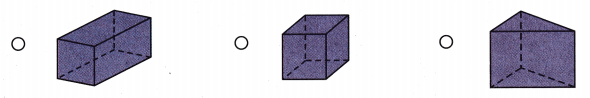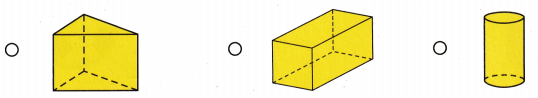Refer to our Texas Go Math Grade 1 Answer Key Pdf to score good marks in the exams. Test yourself by practicing the problems from Texas Go Math Grade 1 Module 15 Assessment Answer Key.

VocabularyQuestion 1.
Color the cube with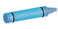(p.558)Question 2.
Color the cone with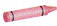(p.552)Question 3.
Color the cylinder with(p.552)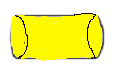Concepts and Skills

Question 4.
Circle the objects you could trace to draw the shape. (TEKS 1.6.E)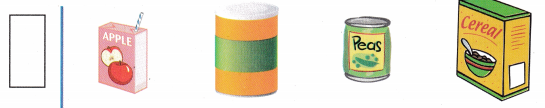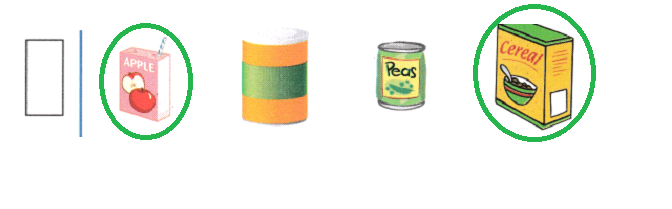Question 5.
Circle the object below that has only a curved surface. (TEKS 1.6.E)

Question 6.
Draw a line under the objects below that have both curved and flat surfaces. (TEKS 1.6.E)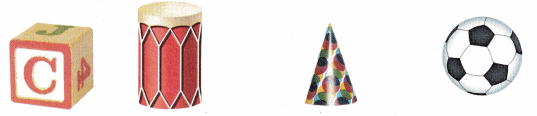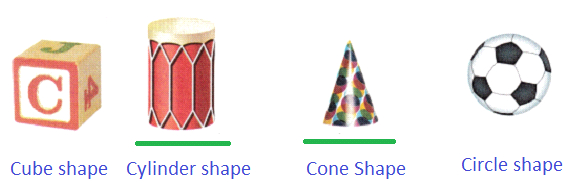Explanation:
3D shapes such as cones and cylinders have a curved surface as well as flat surfaces

Question 7.
Which number completes the sentence?
A rectangular prism has ________ faces. (TEKS 1.6.E)
(A) 8
(B) 6
(C) 4

Explanation:
A rectangular prism has six faces, two at each end and four around.

Question 8.
Which shape can you draw if you trace around a flat surface of a cube? (TEKS 1.6.E)We get the above shape if we trace around a flat surface of a cube.

Question 9.
Which solid has 6 faces? (TEKS 1.6.E)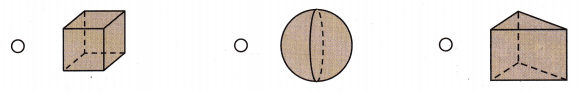A cube is a three – dimensional shape that has six faces.

Question 10.
Which solid has 6 vertices? (TEKS 1.6.E)